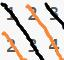# [NOIP2009普及]道路游戏 题解# 题目描述

1. 游戏从小新第一次购买机器人开始计时。
2. 购买机器人和设定机器人的行走次数是瞬间完成的，不需要花费时间。
3. 购买机器人和机器人行走是两个独立的过程，机器人行走时不能购买机器人，购买完机器人并且设定机器人行走次数之后机器人才能行走。
4. 在同一个机器人工厂购买机器人的花费是相同的，但是在不同机器人工厂购买机器人的花费不一定相同。
5. 购买机器人花费的金币，在游戏结束时再从小新收集的金币中扣除，所以在游戏过程中小新不用担心因金币不足，无法购买机器人而导致游戏无法进行。也因为如此，游戏结束后，收集的金币数量可能为负。

# 输入输出样例

2 3 2
1 2 3
2 3 4
1 2


5


# 说明

【数据范围】

NOIP 2009 普及组 第四题

# 题解

## 最初的想法

$$dp[i] = \max \{dp[i - t] + g[i][s - 1] - g[i][s - t - 1] - c[s - t]\}$$## 降低时间：单调队列

$$dp[i] = \max \{dp[i - t] - g[i][s - t - 1] - c[s - t]\} + g[i][s - 1]$$

# 代码

// Code by KSkun, 2018/2
#include <cstdio>
#include <cstring>
#include <deque>
#include <utility>
typedef std::pair<int, int> PI;

std::deque<PI> que;
int n, m, p, sum, cost, dp, v;

inline int minus(int a, int b) { // return a - b
return ((a - b) % n + n) % n;
}

int main() {
scanf("%d%d%d", &n, &m, &p);
for(int i = 0; i < n; i++) {
for(int j = 1; j <= m; j++) {
scanf("%d", &sum[i][j]);
}
}
for(int i = 0; i < n; i++) {
scanf("%d", &cost[i]);
}
// 处理对角线前缀和
for(int i = 2; i <= m; i++) {
for(int j = 0; j < n; j++) {
sum[j][i] += sum[minus(j, 1)][i - 1];
}
}
// 设置初值表示开始一定要买一个机器人
for(int i = 0; i < n; i++) {
que[minus(i, -1)].push_back(std::make_pair(-cost[i], 0));
}
memset(dp, 0xc0, sizeof dp);
for(int i = 1; i <= m; i++) {
for(int j = 0; j < n; j++) {
dp[i] = std::max(dp[i], que[minus(j, i - 1)].front().first + sum[minus(j, 1)][i]);
}
for(int j = 0; j < n; j++) {
while(!que[minus(j, i - 1)].empty() && i - que[minus(j, i - 1)].front().second >= p) {
que[minus(j, i - 1)].pop_front();
}
v = dp[i] - sum[minus(j, 1)][i] - cost[j];
while(!que[minus(j, i - 1)].empty() && que[minus(j, i - 1)].back().first <= v) {
que[minus(j, i - 1)].pop_back();
}
que[minus(j, i - 1)].push_back(std::make_pair(v, i));
}
}
printf("%d", dp[m]);
return 0;
}